In another section a discussion of room modes and examples of how different sources performed in a specific
room was presented. The emphasis there was on how the frequency response varied with source position and
type. Here we shall take a closer look at dipole, cardioid and monopole woofers using an abbreviated
mathematical description of room modes, and examine how the in room response is "built up" from each excited
mode and the implications regarding transient behavior. The simplified aspects of the mathematical formulation
are only for clarity and do not alter the results. In its simplest form the pressure at any frequency,
ω, at ant point,
r=(x,y,z), in a room resulting from a source placed at ro, can be expressed as

p(ω,r|ro) ~  S(ω) ∑ Φn(rn(ro) /(ηn^2 - k^2)                                                        (1)

Here, S(ω), represents the source strength, Φn(r) and Φn(ro) are eigenfunction of the room evaluated at positions
r and ro, (defined below), ηn are the eigenvectors of the room and k is the wave number, ω/c. What this equation
says is that the pressure at a frequency,
ω, at any point, r, in the room resulting from a source at ro is the sum
over n of the product of the n-th eigenfunction evaluated at
r and ro divided by the difference between the
square of the n-th eigenvalue and the wave number, k. Thus, the pressure at any frequency has contributions
from all n modes with the magnitude of the contribution from the n-th mode being dependent on the difference
between the n-th eigenvector and the wave number. If η
n equals k a resonance peak in the pressure can occur.
(Note: in reality the eigenvectors are complex number with real and imaginary parts and the amplitude of a
resonance mode is limited by the magnitude of the imaginary part.). Additionally, as k becomes larger than η
n the
contribution to the pressure from that mode decreases rapidly. Thus, at low frequency where the modes are
widely spaced, the major contribution to the pressure at any frequency is most strongly dependent on the modes
close to the frequency in question. Also note that if eithe
r Φn(r) or Φn(ro) is equal to zero the contribution to the
pressure from that mode will be zero with the listener and source so positioned.

In the above expression for p the index, n, is actually a 3-dimensional index, n = n
x, ny, nz associated with the x, y
and z coordinate directions (length, width and height of the room). The room modes occur at modal frequencies
given as

M(nx,ny,nx) = (c/2)[ (nx/Lx)^2 + (ny/ Ly)^2 + (nz/Lz)^2 ] ^(1/2)                            (2)

where Lx, Ly and Lz are the room length, width and height and each of the indices nx, ny, nz, goes form 0 to
infinity. When any two of the indices are held at zero the modes are referred to as
axial modes in the direction of
the nonzero index. When only one index is held at zero the modes are referred to a
tangential modes in the
plane defined by the directions of the two nonzero indices. When all three indices are nonzero the modes are
referred to as
oblique modes. At low frequency we need only consider the first few modes in each direction.

The simplest form of the eigenfunction for a coordinate with origin is a room corner is the product of cosine
functions in the x,y and z directions;

Φ(r) = cos(π nx X/Lx)  cos(π ny Y/Ly)  cos(π nz Z/Lz)                                        (3)

and the eigenvectors are given as

ηn^2= (π nx /Lx)^2 + (π ny /Ly)^2 +  (π nz /Lz)^2                                              (4)

These forms are for room with perfect rigid wall. When the wall have nonzero admittance the become more
complicated, though similar in character. If we consider the length of the room to be the X direction then when n
y
and n
z are zero M(nx,0,0) are axial modes associated with the room length. When nx is zero the modes M(0,ny,nz)
are the tangential modes in the perpendicular to the X direction. These modes all lie is planes orthogonal to the
room axis, that is in planes parallel to the back wall of the room.

The above relationships apply in general to the placement of a single source in a room. If multiple sources are
present then the pressure at any point is the vector sum of the pressures from the individual sources. If the
sources are arranged to form a dipole the result is,

p(ω,r|ro) ~ S(ω) ∑ [Φn(rn(ro+d/2) - Φn(rn(ro-d/2)] /(ηn^2 - k^2)

~ S(ω) ∑ Φ
n(r) [Φn(ro+d/2) - Φn(ro-d/2)] /(ηn^2 - k^2)                             (5)

where d is the dipole separation and the subtraction is introduced because the phase of the rear source is
inverted. The terms highlighted in blue show that the excitation of a mode by the dipole is dependent on the
difference between the eigenfunctions at the front and rear source positions.

It is also of interest to examine the modification of the form of equation 5 for a cardioid woofer.  When we
consider a cardioid woofer the equation for the in room SPL becomes,

p(ω,r|ro) ~ S(ω) ∑ Φn(r) [Φn(ro+d/2) - Φn(ro-d/2)exp(-iωTd)] /(ηn^2 k^2)          (6)

which is identical to that of the dipole with the exception of the additional factor, exp(-iωTd ). This factor is
introduced to account for the additional time delay which must be applied to the rear source to generate a
cardioid response,

Td =c/d                                                                                                                                      (7)

where c is the speed of sound.

We are now in a position to examine how different sources couple to room modes. We shall examining a dipole in
detail. Once we understand how the individual sources of a dipole couple to the room modes we will recognize
that a monopole is a single source of the dipole and for the cardioid the individual sources couple as the dipole
but sum differently at the listening point due to the additional delay.

Consider the schematic of a room as shown below. The colored surfaces represent the room walls. The blue and
red dots are the sources of a dipole or cardioid positioned at r
o which are separated by a some distance, d, from
each other. The gray dot represents a monopole at r
o. The dipole and cardioid are aligned along the room x
axis, as shown. The gray surface represents a plane in which modes tangential to the dipole axis would lie.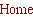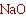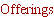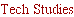Tech Design.....
___________________________

The truth about Cats and Dogs, and Mice; a story of dipole,
cardioid and monopole woofers
.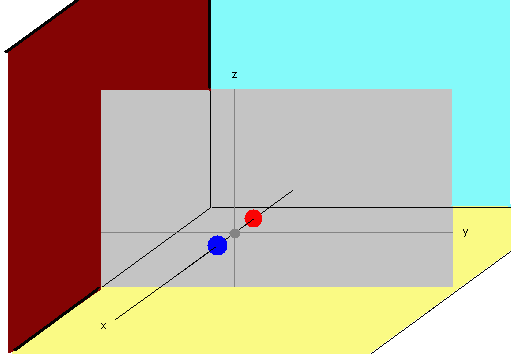Now let us consider as an example the axial M(3,0,0) mode in the direction of
the dipole axis. The eigenfunction of this axial mode given as
cos(3 π x / Lx ).
The positions (values of x) where the cosine is zero are pressure nodes which
are also velocity anti-nodes. The positions where the cosine is a maximum or
minimum are pressure anti-nodes which are also velocity nodes. In the top figure
at the left the dipole/cardioid is positioned at
ro such that they are center at a
pressure node. Since the separation is d, the front and rear sources are
displaced +/- (d/2) from the node. As a result, the values of the eigenfunction at
the front and rear source position are equal in magnitude but opposite in sign.
But for the monopole source placed at
ro the value of the eigenfunction is zero.
The result is that the dipole and cardioid will excite this mode while the monopole
will not. However, the contribution to the in room SPL at any point,
r, depends
not only on whether the node is excited but also on the value of the
eigenfunction at the observation point,
r. As given by equation 1, 5 or 6 above,
the SPL contribution at
r is a function of the product of the value (magnitude) of
the eigenfunction at
r and at the source positions. For the monopole this
product is zero for all
r since the eigenfunction at ro is zero. For the dipole and
cardioid, while different from each other, there will be a position dependent
contribution form this mode to the in room SPL. It is of particular interest to note
that for the dipole this mode will contribute to the in room SPL even for a
listening position 90 degree off the dipole axis where in free space a null would
be present. This is because although the front and rear sources are out of
phase, the sign of the eigenfunction at the front and rear source also inverted
making the net SPL contribution form each source of the same sign.

Looking at the 2nd figure from the top we find the monopole positioned at a
pressure maximum (velocity node). The monopole will excite this mode. For the
dipole and cardioid each source will excite the mode equally, However, since the
dipole sources are out of phase, according to equation 5, regardless of the
listening position, the contributions from the front and rear sources cancel
yielding no net contribution to the in room SPL. This is not the case for the
cardioid due to the additional delay applied to the rear source. Thus, while
different, both the cardioid and monopole will have position dependent
contributions to the in room SPL.

The third figure show the situation when the sources are positioned away from
either a pressure or velocity node. In this case the individual sources of the
dipole and cardioid will excite the mode to different levels. With such placement
monopole, dipole and cardioid will all yield a position dependent contribution to
the in room SPL for that excited mode, even at 90 degrees off axis.

The last figure shows a special case when one source of a dipole or cardioid is
positioned at a pressure node. In the figure the rear source is so positioned.
The result is that the dipole or cardioid would now behave as a monopole since
the rear source would not contribute to the in room SPL for this mode.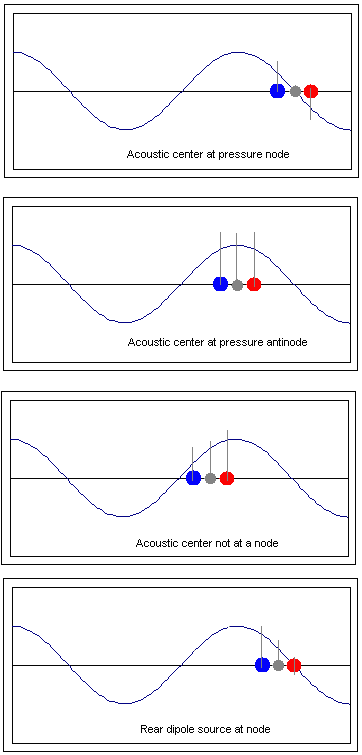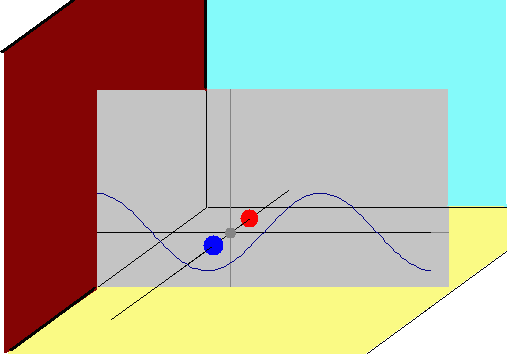Now let us consider happens when we look at a
mode perpendicular to the dipole axis, for example
the
M(0,3,0) mode. This mode is that of a standing
wave from side to side. If the dipole axis remains
parallel to the side walls then the position,
ro, for the
front and rear sources of the dipole will be (x
f, yf, zf)
and (x
r, yr, zr), and yf = yr, with result that the
eigenfunction for this mode will have the same value
for the front and rear sources. In fact, this will be the
case for all modes in the y direction,
M(0,ny,0). If the
dipole axis is also parallel to the floor and ceiling z
f =
z
r as well with further consequence that all the
modes given as
M(0,ny,nz) will have the same value
at the front and rear source position. Looking again
at equation 5 we see that regardless of the listening
position,
r, there will be no contribution to the in
room SPL by any of these modes since,
n(ro+d/2) - Φn(ro-d/2)] = 0                                                                                                                (8)

That these modes are not excited does not mean that the in room SPL at a modal frequency will be the free field value. Remember that
equation 5 states that the in room SPL at any frequency is composed of the sum of the contributions from all the modes with the relative
magnitude of the contributions determined by 1/(F
m^2 - F^2)  where Fm is the modal frequency and F is the frequency where the SPL is
to be evaluated. Since at low frequency the frequency spacing of the modes is fairly wide, the major contribution to the room SPL at any
frequency close to a modal frequency is strongly related to how the mode(s) closest to that frequency are excited. Since the modes
orthogonal to the dipole axis do not contribute to the SPL when the dipole axis is aligned with the room axis there is a possibility that the
low frequency response will have significant nulls near the frequencies of these tangential modes.

When we look equation 6 for the cardioid we see that the term, exp
(-iωTd), multiplying the rear source introduces a frequency
dependent phase shift. Although the magnitude of the eigenfunctions at
ro = +/- d/2 remain equal as for the dipole, this phase shift
result in a none zero sum with the result that the cardioid will produce an SPL contribution form the modes which are perpendicular to
the axis of the cardioid.

These considerations allow us to make the following summary for the three different source types with regard to which modes can
contribute to the in room SPL and under what conditions.

Dipole:

Consequences of source position (independent of orientation):

There will be no contribution to the in room SPL from an axial mode aligned with the dipole axis when dipole is centered at a pressure
node anti-node (velocity node) for that mode.

Additional consequences due to directionality (orientation):

There will be no SPL contribution from any mode in the direction orthogonal to the dipole axis.

Monopole:

Consequences of source position:

There will be no SPL contribution from a mode when placed at a position where that mode has a pressure node.

Being omni directional, a monopole has no consequences arising from directivity.

Cardioid:

Consequences of source position:

In general a cardioid is not limited by position alone with regards to modal contributions to the SPL.

There will be no SPL contribution from a mode when placed at a position where that mode has a pressure node if it is also oriented such
that the mode is orthogonal to the axis of the cardioid.

These considerations concerning positioning indicate that a cardioid woofer may be least sensitive to placement while a dipole will be
the most sensitive, with regard to which modes contribute to the in room SPL. The dipole will also show sensitivity to orientation. The
sensitivity of the dipole to orientation could be viewed a benefit or a detriment. For example, if we consider a full range speaker system
with dipole woofer, then orientation of the speaker for optimal imaging through the mid range may be contrary to the optimum orientation
for bass response.

The view of which woofer format is the ideal will also depend on the different schools of though regarding low frequency, in room
reproduction. One school favors the idea that the best low frequency response can be achieved by exciting as may of the room modes
as possible. This approach can be accomplished with any of the woofer systems but may slightly favor the cardioid due to its relative
lack of sensitivity to position or orientation. Another school favors exciting fewer room modes. This would tend to favor dipole woofers
since they can be positioned in a manor to minimize the number of excited modes.
Listening Position:

Regardless of source type, it must be recognized that which and by how much a mode is excited is not the sole factor in determining the
SPL at the listening position. As shown by equations 1, 5 and 6, an excited mode will not contribute to the in room SPL at the listening
position if the listening position is at a pressure node, regardless of source type. This is because if the listening position is at a pressure
node Φ
n(r) will have zero magnitude and regardless of the excitation of the mode by the source the SPL contribution from that mode will
multiplied by Φ
n(r) = 0.

The DC mode:

There is one additional consideration which must be given to a dipole woofer. This is the behavior between the DC mode and the first,
lowest frequency room mode, the fundamental mode. The DC mode is
M(0,0,0). As seen from equation 3 the DC mode equals1.0
everywhere in the room, regardless of position. The DC mode is a pressure mode. Equation 5 tells us that irregardless of the strength
of the dipole sources the dipole can not excite the DC mode and there can be no contribution to the SPL from this mode. Cardioids and
monopoles can excite the DC mode. The difference in this behavior means that monopoles can cardioids can benefit from room
pressurization effects where as a dipole can not. In fact, if a dipole woofer is placed and orientated so as to excite as many room modes
as possible, then this is the only significant difference between a dipole and the other two source types with regards to excitation of
modes
. Further discussion of room pressurization may be found here.

Building the Room SPL

We now have an idea of the way in which different sources may excite different room modes and the role of the listening position in
determining how an excited mode will contribute to the low frequency response at a given position. It may also be beneficial to develop
an deeper understanding of how each mode contributes to the to the response. For example, if a specific mode is not excited this does
not mean that the SPL around that modal frequency will be the free field value, nor does it mean there will necessarily be a deep null at
that frequency. In the low frequency range where the modal spacing is relatively wide this may be the case, but as the frequency
increases the modal density also increases and the consequence of not exciting a mode becomes less significant. For example, the
figure below shows the room modes for a 5 M wide by 7 M long by 2.44 M high (8') room. The first floor to ceiling modes occurs at
approximately 70 Hz and is shown in black. It is the only mode in the vertical direction below 100 Hz, and, as can be seen, there are
other modes closely spaced around this mode. Thus, if this mode is not excited, or excited only weakly, the SPL around 70 Hz will have
contributions from other modes. Such may not be the case if the first mode in the side to side direction, at 34 Hz shown in fuchsia, is not
excited. If this mode is not excited we should not be surprised to expect a dip in the in room response in this frequency range.
Fourier transformation of this contribution will be the contribution to the transient behavior from that mode at the listening position.
Since the steady state frequency response is the sum of the steady state contributions from all the modes, it follows that the transient
response is the inverse Fourier transformation of this sum.

First we need to understand some properties of Fourier transformations. If a frequency response is represented as a sum,

H(ω) = A(ω) + B(ω),                                                                                                                 (9)

where A(ω) and B(ω) are components of the frequency response, H(ω), then the impulse response,

h(t) = iF [H(ω)] = iF [A(ω) + B(ω)] = iF [A(ω)] + iF [B(ω)] = a(t) + b(t)                                   (10)

where iF  indicates the inverse Fourier transformation. Likewise, if the frequency response is the product of two
separate responses,

H(ω) = A(ω) B(ω),                                                                                                                    (11)

then

h(t) = iF [H(ω)] = iF [A(ω) B(ω)] =  a(t) ¤ b(t)                                                                          (12)

where the symbol ¤ indicates convolution. Thus, for the pressure response, since

P(ω,r|ro) = ∑m P(ω,r|ro)m ~  S(ω) ∑m Hm(ω,r|ro)                                                                          (13)

then,

p(t,r|ro) = iF [ P(ω,r|ro)] =∑m  iF [ Pm(ω,r|ro)] ~ sω(t) ¤m hm(t,r|ro)                                              (14)

where sω(t) is the impulse response of the source. Thus we see that the impulse response at any point in the room is the convolution
of the impulse response of the source with an impulse train that is the sum of the impulse responses of the transfer functions between
source and listening position for each mode. Ultimately what this tells us is that the transient response at low frequency is directly
related to the frequency response. Just as the frequency response changes with listening position for a fixed source position, so must
the transient response. It follows that the only difference between source types with regard to transient response must be due to
differences in frequency response, either in amplitude, phase or both. If we consider two different frequency response profiles at a
listening position, one generated by a dipole positioned so as not to excite the
M(0,ny,nz) modes and the second by another source
which will excite these modes, then assuming both response profiles can be equalized to yield the same response at the listening
position it follows that, if the response at the listening position can be reduced to minimum phase, then the transient response at the
listening position must be independent of source type. If the response is non-minimum phase then if the amplitude response is
identical any differences in the transient response are a direct result of differences in the phase response.

So, how can we examine the critical issue of whether or not the in room response of a woofer can be reduced to minimum phase?
Recall that the contribution to the frequency response from each mode is that of a 2nd order low pass filter with cut off frequency at
the resonant frequency of the mode, Q determined by the room damping, pass band amplitude proportional to
|Φn(rn(ro) /ηn^2|, and
phase (zero or inverted) determined by the sign of Φ
n(rn(ro). As a result the impulse response of a mode can be expressed as
An(r|ro) x ln(t), where ln(t) is the impulse response of a 2nd order low pass filter with cut off frequency and Q corresponding to the
mode and the factor
An, sets the passband amplitude and phase. The impulse response at any position in the room is thus,

h(t,r|ro) = n ( An(r|ro) x ln(t) )                                                                                                        (15)

While there are cases where it can be shown that an impulse response composed of the sum of a series of impulses is minimum
phase, in general this is not the case. Thus, in general we must conclude that the response at the listening position is most likely not
minimum phase and the transient response will be different for different sources even after equalization to an common amplitude
response. Furthermore, if the listening position is changed and the response equalized for this new position the transient response
may be different even if the source type does not change.

This leads to even more confusion with regards to how to best achieve accurate low frequency response in a listening room under the
assumption that the response is non-minimum phase. Previously is was shown that the different source types are more or less
sensitive to position and orientation with regard the number modes excited, but how does this relate to the variation of transient
response with position and accuracy? One would think that is the number of modes excited is insensitive to placement then it would
follow that the transient response would also be less sensitive to placement. But this says nothing about the accuracy of the transient
response. Looking at equation 13 we might conclude that the most accurate transient response would be obtained when the fewest
modes were excited, that is, when the impulse train is the summation of the fewest modal impulses with non zero amplitude. This may
well be the case. If so, it would seem to create a dilemma; a source which excites the most modes should be least sensitive to position
yielding a frequency and transient response which may be more consistent over a wider range of listening and source positions while,
on the other hand, a source which excites fewer modes could potentially yield more accurate response for specific source and listener
location, but would likely show greater variation with changes in position.

To shed some light on this a number of calculations were done using the same room and listening position specifications with
monopole, dipole and cardioid sources. In each case the in room frequency response at the listening position was computed.
This
amplitude response was equalized to yield an idealized response of a woofer system with a 20 Hz, 2nd order, Q = 0.5
high pass response and a 120 Hz, 4th order Butterworth low pass response at the listening position
. Then the response of
the equalized system to a 10 msec wide pulse was computed.

The first set of computations was for a monopole, dipole and cardioid where only the modes in the direction of the main room axis
(front to back) were included. The dipole and cardioid axes were aligned with this direction. This is obviously a very unrealistic, one
dimensional calculation, basically representative of a situation where the room height and width are much smaller than the room
length; a duct of rectangular cross section, if you like. But it is useful to see the limitations of a one dimensional analysis of room
modes.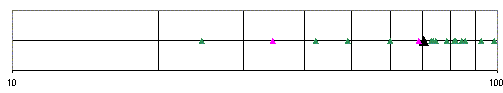Room mode for a 5M x7M x 2.44M room
We must recall that the in room SPL can be considered as being built from sum of the responses of the excited modes. Since dipoles
and cardioids are constructed from monopole sources we need only examine how the SPL is build from these modes for a monopole
to deepen our understanding. Form equation 1 we recognize that for each mode we can express the source to listing position transfer
function as,

Hn(ω,r|ro) =  Φn(rn(ro) /(ηn^2 - (ω/c)^2)

While it may not be apparent, this is actually the transfer function of a 2nd order low pass filter with corner frequency equal to the
modal frequency and Q determined by the imaginary part of η
n. The imaginary part of ηn is the damping of the mode. For example, Hn
for the mode M(1,0,0) in a 5 x 7 x 2.44 N room with moderate damping would appear as shown below for a specific source and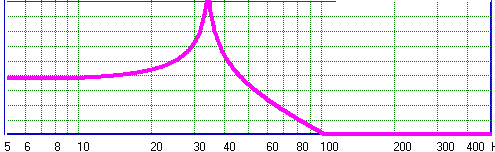listener location. If this were the only mode excited
and the source had flat free filed response, the figure
to the left would represent the in room low frequency
response at the prescribed listening and source
positions. Every excited mode contributes to the in
room SPL at all frequencies with the contribution from
each mode being that of the 2nd order low pass filter
with cut off frequency at the resonant frequency of
the mode, Q determined by the room damping
of the mode, and a pass band amplitude proportional to |Φn(rn(ro) /ηn^2|. The phase response of each mode is minimum phase with
either normal or inverted polarity, as  determined by the sign of Φ
n(rn(ro).The in room response can thus be though of as being the
summed response of an infinite number of low pass filters with cut off frequency given by the modal frequencies. As η
n becomes large
the pass band amplitude decreases, thus higher frequency modes contribute less and less to the SPL at low frequency response.

Transients:

Looking at the in room response in this manner has interesting implications with regard to the in room transient behavior. The
transient response at a given listening position for a given source position is dependent on how each excited room mode responds in
time. If we know the steady state behavior of a specific mode as a function of frequency we know the contribution of that mode to the
frequency response at the listening position. Assuming the room/source represents a linear, time invariant system, the inverse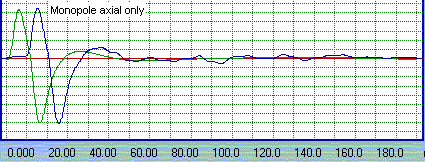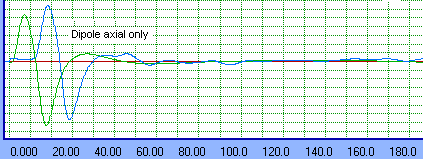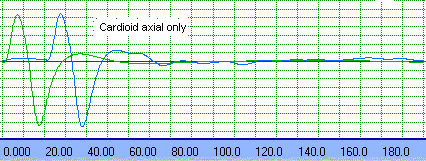The figures to the left show the results of these one
dimensional computations. In all cases the green curve is the
response to a 10 msec impulse for a band pass system with
the same high pass and low pass characteristics as the woofer
system equalization target. This would be the response of the
woofer system in free space, assuming the woofer is a
minimum phase device. The blue curve is the impulse for the
equalized in room response. Aside from the time offset and
possibly polarity, this response should be identical to the
green curve if the in room response is minimum phase.
Apparently, when limited to a one dimensional modal analysis
none of the source types exhibit a purely the minimum phase
pulse response, although they all  very close, at least for the
woofer and listener positions chosen.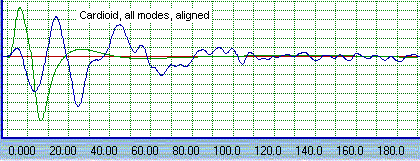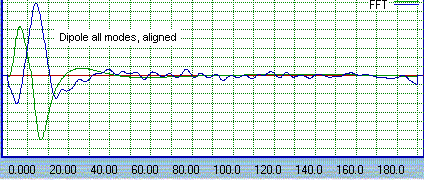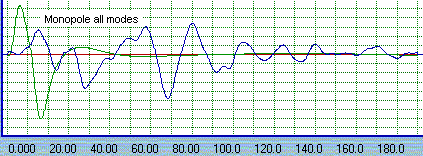The next series of figures at the right show the result when all
room modes are considered. The cardioid and dipole axes
remain aligned with the room's main axis. Here we see that the
monopole and cardioid response changes dramatically as a
result of the introduction of the additional modes. However, the
dipole response isn't all that different from the case above if the
inversion is ignored. This is to be expected since with the dipole
axis aligned as such, the axial modes in the directions of the
room height and width and the tangential modes perpendicular to
the dipole axis are not excited. Thus, the only difference between
this and the previous case for the dipole is the introduction of the
oblique modes. However, other placements have  shown the
response of all the sources to suffer more or less degradion from
the ideal response. Recall that in each case the amplitude
response is equalized to the ideal amplitude at the listening
position.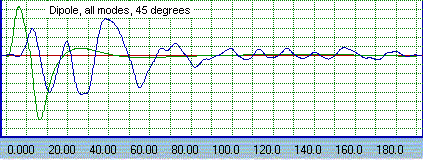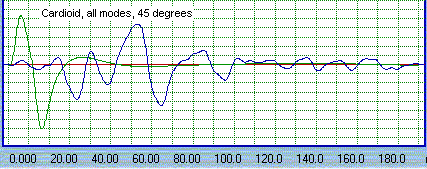This last set of figures shows the pulse response when the dipole
and cardioid are rotated so that the axis is at 45 degrees to the
room main axis. When positioned in this manor the dipole is
capable of exciting all the modes with the exception of the axial
modes in the floor to ceiling direction. The change in position of
the front and rear sources due to the rotation also means that the
modes which were previously excited will be excited differently.
For the cardioid there is no change in the modes which may be
excited, however since the front and rear sources are in slightly
different positions the modes are excited differently. For the
monopole, obviously since orientation doesn't make a difference,
there is no change.

In all cases we see that the pulse response of the woofer is a far
cry form the ideal pulse response shown in green. There is also
no evidence that the pulse response form any one the source is
better than any other.
Form looking at the pulse response behavior we can conclude several things. First, a one dimensional analysis of the response of the
different woofer systems, when equalized to a common response at the listening position, is clearly inadequate. Second, the deviation of
the pulse response form the ideal impulse clearly shows that the in room response of any of the woofers is not minimum phase. Third,
while there may be some benefit to a dipole woofer, with regard to transient response, if the dipole axis is aligned with a room axis such
benefit is lost if the dipole is rotated towards the listening position, as may be required of a full range speaker system for optimal
midrange imaging. Fourth, we must realize that the results above are for a closed, rectangular room in which the response at the
listening position has been equalised to a common band pass response. This is a highly unlikely scenario since most home listening
rooms, while they may be nominally rectangular, will usually have openings to other rooms, lofts, etc, and the equalization required to
achieve the type of response emulated in these calculations would not be present. Even if equalization were applied it would most likely
be applied in a manner to optimize the response over a wider listening area than at a single position.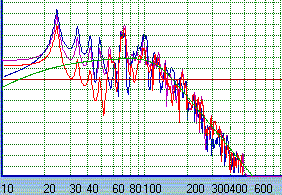With that in mind this figure is presented to show the unequalized response which
would be expect at the listening position. Red is the monopole, blue the dipole and
violet the cardioid.
But wait! There is one positioning that is typically
overlooked. Whats if the  woofer is placed very close to
the listening position? If the source and listener are at
the exact same placement the room transfer function is
as shown to the left. The frequency response is flat
and minimum phase. Thus if the  woofer response was
superimposed on the room transfer function there
would be no amplitude or phase distortion other than
that of the woofer as in the free field. (The slight
wiggles in the curve to the left are a result of
consideration of only a finite number of modes in the
summation given by equation 1. )

When we place the woofer slightly away from the
listener the response is as shown in  the lower figure.
Here the woofer is positioned just behind the listening
position. While the response is not flat, it remains
minimum phase. as is observed by the overlay of the
green, calculated phase and the dark blue  minimum
phase constructed form the amplitude.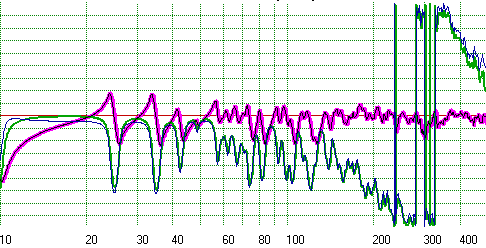Again, this is only the room-listener transfer function. But when the woofers free field response is superimposed on it, it is apparent
that the response could be equalized to correct the irregularities while retaining a minimum phase response. The obvious draw back is
that the response can only be optimized of a single listener. Never the less, that listener would experience the most accurate bass
response with transient characteristics similar to that of the woofer is the free field.